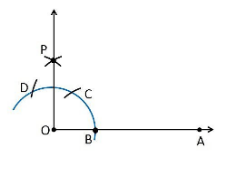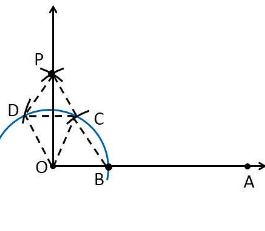Guru

# Construct an angle of 90° at the initial point of a given ray and justify the construction. Q.1

• 0

How i solve the question of class 9th ncert math of Constructions chapter of exercise 11.1 of question no  1. I think it is very important question of class 9th give me the tricky way for solving this question Construct an angle of 90° at the initial point of a given ray and justify the construction

Share

1. Construction Procedure:

To construct an angle 90°, follow the given steps:

1. Draw a ray OA

2. Take O as a centre with any radius, draw an arc DCB is that cuts OA at B.

3. With B as a centre with the same radius, mark a point C on the arc DCB.

4. With C as a centre and the same radius, mark a point D on the arc DCB.

5. Take C and D as centre, draw two arcs which intersect each other with the same radius at P.

6. Finally, the ray OP is joined which makes an angle 90° with OP is formed.Justification

To prove ∠POA = 90°

In order to prove this, draw a dotted line from the point O to C and O to D and the angles formed are:From the construction, it is observed that

OB = BC = OC

Therefore, OBC is an equilateral triangle

So that, ∠BOC = 60°.

Similarly,

OD = DC = OC

Therefore, DOC is an equilateral triangle

So that, ∠DOC = 60°.

From SSS triangle congruence rule

△OBC ≅ OCD

So, ∠BOC = ∠DOC [By C.P.C.T]

Therefore, ∠COP = ½ ∠DOC = ½ (60°).

∠COP = 30°

To find the ∠POA = 90°:

∠POA = ∠BOC+∠COP

∠POA = 60°+30°

∠POA = 90°

Hence, justified.

• 0